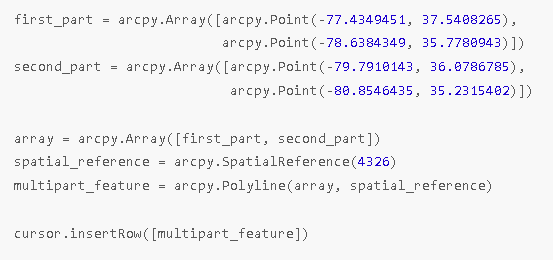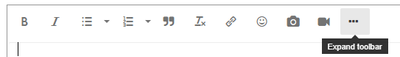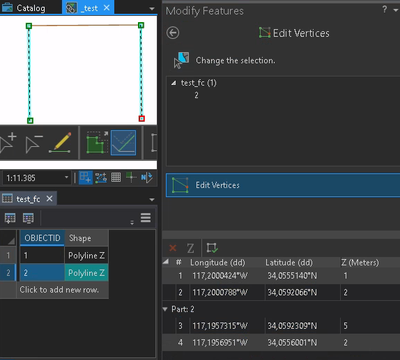# Creating multipart polyline with Z values

105
1
08-14-2022 07:29 AMby
New Contributor II

Trying to create multipart polyline from array of arrays (arcpy.Polyline containing multiple arcpy.Array (each is a singlepart line)) or list of coords. Tried it in multiple ways.Inserting a list of 3D coords into Z-enabled feature class as shown in https://pro.arcgis.com/en/pro-app/latest/arcpy/get-started/writing-geometries.htm class only worked for singlepart features for me.

Creating multipart polyline without Z values also works as also shown in Write Geometries:This also didn´t work after I added z-coord into the points.

Created multipart polyline without z-coord, inserted it into Z-enabled feature class. Tried to update z-coords using script similar to https://community.esri.com/t5/python-questions/change-z-coordinates-of-vertexes-by-updatecursor/m-p/... but applied to ArcGIS Pro 2.9, the script can run without errors. After I change z-coord of a point, .Z property prints correct z-coord. But after I create a new multipart polyline from points with correct Z-coord and update the row with it, Z-coords of all updated polyline points are equal to 0.

Does anyone know how to solve this?

Tags (4)
1 Solution

Accepted SolutionsbyMVP Regular Contributor

Code screenshots are hard to work with, because we can't easily test what you did. To post code as text:Passing a simple list of coordinate tuples to the InsertCursor seems to be a shortcut that is only valid for singlepart features. Personally, I always create an arcpy.Geometry object and pass that.

``````test_fc = arcpy.management.CreateFeatureclass("memory", "test_fc", "POLYLINE", has_z="YES", spatial_reference=4326)

coordinates = [
[-117.2000424, 34.055514, 1],
[-117.2000788, 34.0592066, 2],
[-117.1957315, 34.0592309, 5],
[-117.1956951, 34.0556001, 2],
]

with arcpy.da.InsertCursor(test_fc, ["SHAPE@"]) as cursor:
points = [arcpy.Point(c, c, c) for c in coordinates]
line = arcpy.Polyline(arcpy.Array(points), has_z=True, spatial_reference=4326)
cursor.insertRow([line])````````````multipart_coordinates = [
[coordinates, coordinates],
[coordinates, coordinates],
]

with arcpy.da.InsertCursor(test_fc, ["SHAPE@"]) as cursor:
parts = arcpy.Array([
arcpy.Array([
arcpy.Point(p, p, p) for p in part
])
for part in multipart_coordinates])
line = arcpy.Polyline(parts, has_z=True, spatial_reference=4326)
cursor.insertRow([line])``````Have a great day!
JohannesbyMVP Regular Contributor

Code screenshots are hard to work with, because we can't easily test what you did. To post code as text:Passing a simple list of coordinate tuples to the InsertCursor seems to be a shortcut that is only valid for singlepart features. Personally, I always create an arcpy.Geometry object and pass that.

``````test_fc = arcpy.management.CreateFeatureclass("memory", "test_fc", "POLYLINE", has_z="YES", spatial_reference=4326)

coordinates = [
[-117.2000424, 34.055514, 1],
[-117.2000788, 34.0592066, 2],
[-117.1957315, 34.0592309, 5],
[-117.1956951, 34.0556001, 2],
]

with arcpy.da.InsertCursor(test_fc, ["SHAPE@"]) as cursor:
points = [arcpy.Point(c, c, c) for c in coordinates]
line = arcpy.Polyline(arcpy.Array(points), has_z=True, spatial_reference=4326)
cursor.insertRow([line])````````````multipart_coordinates = [
[coordinates, coordinates],
[coordinates, coordinates],
]

with arcpy.da.InsertCursor(test_fc, ["SHAPE@"]) as cursor:
parts = arcpy.Array([
arcpy.Array([
arcpy.Point(p, p, p) for p in part
])
for part in multipart_coordinates])
line = arcpy.Polyline(parts, has_z=True, spatial_reference=4326)
cursor.insertRow([line])``````Have a great day!
Johannes